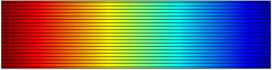# アプリケーションギャラリ

#### Gravity and Boundary Conditions

Application ID: 2152

The influence of gravity on the flow pattern is often an important issue when modeling flow in fluids with variable density. You can account for this influence in the model equations by adding, to the momentum balances, the volume force.

This model shows a simple example of the implementation of boundary conditions when volumetric forces are included in the momentum balances. This can be used to account for hydrostatic pressure and to account for density variations in free convection problems.This model example illustrates applications of this type that would nominally be built using the following products: